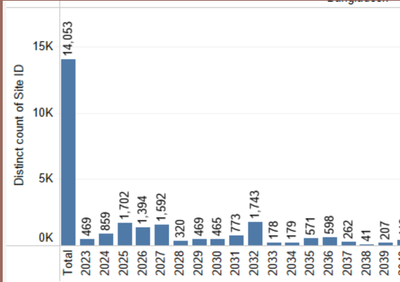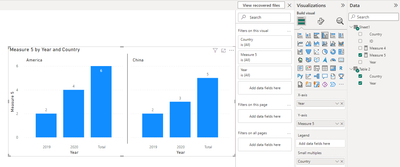cancel
Showing results for
Did you mean:

Fabric is Generally Available. Browse Fabric Presentations. Work towards your Fabric certification with the Cloud Skills Challenge.New Member

## Adding Grand total to column chart calculating distinct count

Hi,

I am ttrying to do up a bar chart for countries across years with the distinct count of IDs as the value. I am also trying to add a grand total column at the side of each chart, looking something like this:How do i go about doing this?

I have attached a very simplified version fo my data, bearing in mind the total should work and be accurate when factoring in slicers and filters.

Thank you for the help!

1 ACCEPTED SOLUTIONCommunity Support

Hi @ipmh97 ,

According to your description, here are my steps you can follow as a solution.

(1) My test data is the same as yours.

(2) We can create a table and measures.

``````Table 2 = var a=SUMMARIZE(Sheet1,[Year])
var b={"Total"}
var c=SUMMARIZE(Sheet1,[Country])
return GENERATEALL(UNION(a,b),c)``````
``````Measure 4 = var a=SUMMARIZE(FILTER('Table 2',[Year]<>"Total"),'Table 2'[Year])
return IF(SELECTEDVALUE('Table 2'[Year])<>"Total",CALCULATE(DISTINCTCOUNT(Sheet1[ID]),FILTER('Sheet1',[Year] in a&&[Country] in VALUES('Table 2'[Country]))),0)``````
``````Measure 5 = var a=SUMMARIZE(FILTER(ALLSELECTED('Table 2'),[Year]<>"Total"),[Year],[Country],"Sum",[Measure 4])
return IF(SELECTEDVALUE('Table 2'[Year])="Total",SUMX(FILTER(a,[Country] in VALUES('Table 2'[Country])),[Sum]),SUMX(FILTER(a,[Year] in VALUES('Table 2'[Year])&&[Country] in VALUES('Table 2'[Country])),[Sum]))
``````

(3) Then the result is as follows.Best Regards,

Neeko Tang

If this post  helps, then please consider Accept it as the solution  to help the other members find it more quickly.Community Support

Hi @ipmh97 ,

According to your description, here are my steps you can follow as a solution.

(1) My test data is the same as yours.

(2) We can create a table and measures.

``````Table 2 = var a=SUMMARIZE(Sheet1,[Year])
var b={"Total"}
var c=SUMMARIZE(Sheet1,[Country])
return GENERATEALL(UNION(a,b),c)``````
``````Measure 4 = var a=SUMMARIZE(FILTER('Table 2',[Year]<>"Total"),'Table 2'[Year])
return IF(SELECTEDVALUE('Table 2'[Year])<>"Total",CALCULATE(DISTINCTCOUNT(Sheet1[ID]),FILTER('Sheet1',[Year] in a&&[Country] in VALUES('Table 2'[Country]))),0)``````
``````Measure 5 = var a=SUMMARIZE(FILTER(ALLSELECTED('Table 2'),[Year]<>"Total"),[Year],[Country],"Sum",[Measure 4])
return IF(SELECTEDVALUE('Table 2'[Year])="Total",SUMX(FILTER(a,[Country] in VALUES('Table 2'[Country])),[Sum]),SUMX(FILTER(a,[Year] in VALUES('Table 2'[Year])&&[Country] in VALUES('Table 2'[Country])),[Sum]))
``````

(3) Then the result is as follows.Best Regards,

Neeko Tang

If this post  helps, then please consider Accept it as the solution  to help the other members find it more quickly.Announcements#### Power BI Monthly Update - November 2023

Check out the November 2023 Power BI update to learn about new features.#### Fabric Community News unified experience

Read the latest Fabric Community announcements, including updates on Power BI, Synapse, Data Factory and Data Activator.#### The largest Power BI and Fabric virtual conference

130+ sessions, 130+ speakers, Product managers, MVPs, and experts. All about Power BI and Fabric. Attend online or watch the recordings.Top Solution Authors
Top Kudoed Authors
Users online (3,631)